Monday, September 27, 2021
Home > geometry > SSC CGL Geometry Congruent Triangles Set 1

# SSC CGL Geometry Congruent Triangles Set 1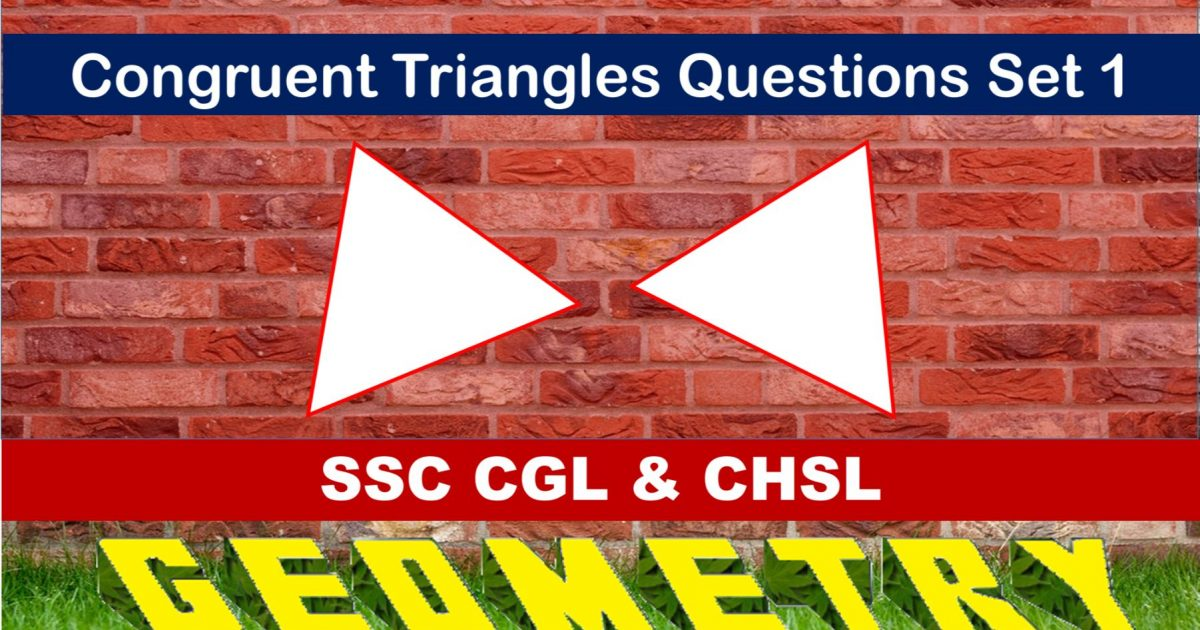SSC CGL Geometry Congruent Triangles Set 1 : Ques No 1

In the figure below, AY ⊥ YZ and BY ⊥ XY such that AY = YZ and BY = XY. If k is the ratio of the lengths of AB and ZX, then the value of k isOptions:

A. 1

B. 1/2

C. 2

D. 2/3

Solution:

SSC CGL Geometry Congruent Triangles Set 1 : Ques No 2

In the figure below, the triangle ABC is an isosceles triangle with AB = AC. X and Y are two points on equal sides AB and AC respectively such that AX = AY. If CX = 5 units, then the value of BY isOptions:

A. 1

B. 3

C. 5

D. 2

Solution:

SSC CGL Geometry Congruent Triangles Set 1 : Ques No 3

In the figure below, if the angles x and y are equal and AB = BC, then which of the following is correct?Options:

A. DF = EF

B. AE = CD

C. BE = BF

D. AF = FC

Solution:

SSC CGL Geometry Congruent Triangles Set 1 : Ques No 4

In the figure below, D is the mid-point of BC. If DL and DM are perpendiculars drawn on AB and AC respectively such that DL = DM, then the value of AB – AC isOptions:

A. 0

B. 1

C. 2

D. 3

Solution:

SSC CGL Geometry Congruent Triangles Set 1 : Ques No 5

In the figure below, ABC is an equilateral triangle, PQ || AC and AC is produced to R such that CR = BP, then which of the following is incorrect?Options:

A. PQ = CR

B. PM = MC

C. BP = PQ

D. BP = PM

Solution:

SSC CGL Geometry Congruent Triangles Set 1 : Ques No 6

In the figure below, AC = BC, ∠DCA = ∠ECB and ∠DBC = ∠EAC. If p = DC / EC and q = BD / AE, then the value of (p + q) is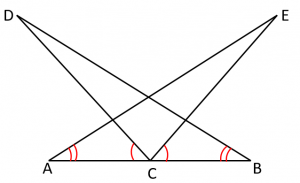Options:

A. 1

B. 2

C. 3

D. 4

Solution:SSC CGL Geometry Congruent Triangles Set 1 : Ques No 7

In the figure below, BD and CE are altitudes. If BE = CD, then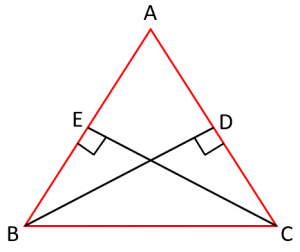Options:

A. AB = BC

B. AE = AC

C. BD = CE

D. None of these

Solution:

SSC CGL Geometry Congruent Triangles Set 1 : Ques No 8

In the figure below, AB = CF, EF = BD and ∠AFE = ∠CBD, then which of the following is incorrect?Options:

A. AF = CB

B. ∠AEF = ∠CDB

C. AE = CD

D. None of these

Solution:

SSC CGL Geometry Congruent Triangles Set 1 : Ques No 9

In the figure below, ∠A = ∠C and AB = BC, then which of the following is incorrect?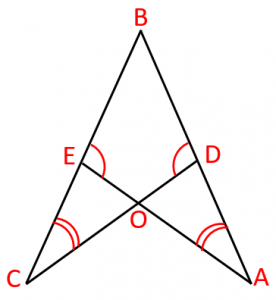Options:

B. BD = BE

C. OA = OC

D. None of these

Solution:

SSC CGL Geometry Congruent Triangles Set 1 : Ques No 10

ABC and DBC are two isosceles triangles on the same base and the vertices A and D are on the same side of BC. If AD is extended to intersect BP at P, then which of the following is incorrect?

Options:

A. ∆ABD ≅ ∆ACD

B. ∆ABP ≅ ∆ACP

C. AP bisects ∠A and ∠D

D. None of these

Solution:

SSC CGL Geometry Congruent Triangles Set 1 : Ques No 11

ABCD is a square, X is a point in the diagonal BD and the perpendicular from B to AX meets AC in Y. Then, which of the following is true?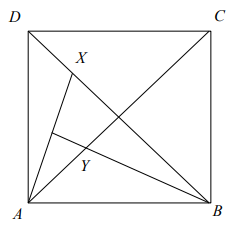Options:

A. ∆AXD ≅ ∆AYB

B. ∆BCY ≅ ∆AXB

C. Angle ADX = Angle AYB

D. None of these